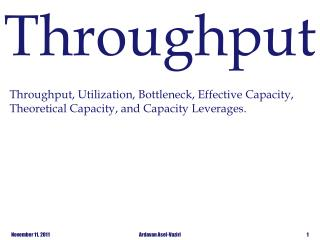Download PresentationThroughput

Throughput - PowerPoint PPT Presentation

Throughput. Throughput, Utilization, Bottleneck, Effective Capacity, Theoretical Capacity, and Capacity Leverages. Flow Rate, Throughput, Takt Time and Capacity. Throughput is the number of flow units per unit of time. Observe the process for a number of periods .I am the owner, or an agent authorized to act on behalf of the owner, of the copyrighted work described.
Download PresentationThroughput

Download Policy: Content on the Website is provided to you AS IS for your information and personal use and may not be sold / licensed / shared on other websites without getting consent from its author.While downloading, if for some reason you are not able to download a presentation, the publisher may have deleted the file from their server.

- - - - - - - - - - - - - - - - - - - - - - - - - - E N D - - - - - - - - - - - - - - - - - - - - - - - - - -
Presentation Transcript
1. Throughput Throughput, Utilization, Bottleneck, Effective Capacity, Theoretical Capacity, and Capacity Leverages. Ardavan Asef-Vaziri

2. Flow Rate, Throughput, Takt Time and Capacity • Throughput is thenumber of flow units per unit of time. • Observe the process for a number of periods. • Measure the number of flow units that are processed per unit of time. • Compute the average number of flow units per unit of time. • Throughput = Average Flow Rate • Capacityis the maximum sustainable flow rate. In periods of heavy congestion, throughput is equal to capacity. • In a synchronized operation: Throughput = Demand < Capacity 2 Throughput Analysis Ardavan Asef-Vaziri, June 2013

3. Flow Rate, Throughput, Takt Time and Capacity Cycle Time is defined in terms of Capacity. Cycle time = 1/Capacity Capacity = 15/hour Cycle Time = 1/15 hour Cycle Time = 4 minutes Takttime derived from the German word “pace”. TaktTime is defined in relation to Demand or Throughput. Demand = 12/hour  Throughput = 12/ hour Takt Time = 1/Demand = 1/(Throughput) = 1/R Takt Time = 1/12 hour Takt Time = 5 minutes 3 Throughput Analysis Ardavan Asef-Vaziri, June 2013

4. Flow Rate, Throughput, Takt Time and Capacity R= Ra  Takt time = 1/Ra Average inter-arrival time = Ta = 1/Ra = 1/R= Average inter- exit time It is not uncommon for managers to state that their machines have takt time of five minutes (1/12 hour). It is wrong. Takt time is a measure of external demand, it has nothing to do with the internal measure of machine capacity. In a synchronized system, Takt time is the time each station has to send one flow unit out to the next station. Chapter 4 was on flow time minimization. Chapter 5 is on throughput maximization. Chapter 4 and 5 are both “time” minimizations. Why? 4 Throughput Analysis Ardavan Asef-Vaziri, June 2013

5. Activity Time, Unit Load, Total Unit Load Operation B Operation A If both are done on Recourse (1) then the theoretical unit load of Resource(1) is 10+5 = 15 min. If Capacity waste factor in both stations is 25% then the total unit load 15/(1-0.25) = 20 min. We refer to this as Tp. It is not important to memorize the theoretical unit load and the unit load. What is important is if we do not consider Capacity Waste Factor then we are talking about the theoretical capacity. If we consider, then we are talking effective capacity or simply capacity. Activity Time = 5 min Activity Time = 10 min

6. Resources, Resource Pools and Resource Pooling Unit Load of a Resource Unit (Tp) – The amount of time the resource works to process each flow unit. This includes a share of all distractions such as maintenance, repair, setup, etc. It is aeffective unit load not the theoretical unit load. 6 Throughput Analysis Ardavan Asef-Vaziri, June 2013

7. Effective Capacity Effective Capacity of a Resource Unit Rp= 1/unit load = 1/ Tp Effective Capacity of a Resource Pool = Rp=c/Tp Bottleneck – The resource pool with the minimum effective capacity. Effective capacity of a process: Effective capacity of the bottleneck. Cross train Claims Supervisor to help Mailroom clerk  Increase Capacity. 7 Throughput Analysis Ardavan Asef-Vaziri, June 2013

8. 100% Utilization is a High Risk Imagine a a freeway where all the cars are driving exactly 65 and the distance between pairs of cars in 1 inch. As long as every one has a speed of exactly 65 that is fine. But can they do that? What happens if one hits the break? How long does it take to clean the freeway. Do cars pass freeway easier when utilization is 1 and they are moving bumper to bumper, or when 50% of the freeway is empty, U = 0.5, or when U = 0.25. How long it takes to clean up accidents in these situations? Never make U of all the sub-processes or activities, and not even a single sub-process = 1 8 Throughput Analysis Ardavan Asef-Vaziri, June 2013

9. Capacity Utilization R = 400 per day. Capacity utilization of a resource pool Up = Throughput/Effective capacity of a resource pool = R/Rp Capacity utilization of the process U= Throughput/Effective capacity of the bottleneck resource pool 9 Throughput Analysis Ardavan Asef-Vaziri, June 2013

10. Cycle Time, Takt Time, Flow Time Day = 480 minutes. Rp = 480 per day Cycle time = 1/Rp CT = 1/480 day CT = 480(1/480) = 1 minute We already knew CT = 1 min. R = 400 per day Takt Time = 1/R TT = 1/400 day TT=480(1/400) = 1.2 minute Flow Time = 1+5+8+2.5 FT = 16.5 10 Throughput Analysis Ardavan Asef-Vaziri, June 2013

11. Unit Load for a Product Mix Billing: Physician claims 60%, Hospital claims 40% Throughput is 400 units per day. Process utilization: U =100% 11 Throughput Analysis Ardavan Asef-Vaziri, June 2013